# R S Aggarwal Solutions for Class 10 Maths Chapter 19 Probability

RS Aggarwal Solutions for class 10 chapter 19 Probability are available here. Class 10, Chapter 19 solutions are prepared by subject experts at BYJU’S based on latest CBSE Syllabus. Students are advised to solve R S Aggarwal Solutions for Maths to sharpen their skills.

This study material is the best resource for the students appearing for the board exams and prepare them for advanced probability problems. Students can download R S Aggarwal Solutions pfd for free to practice offline.

## Download PDF of R S Aggarwal Solutions for Class 10 Chapter 19 Probability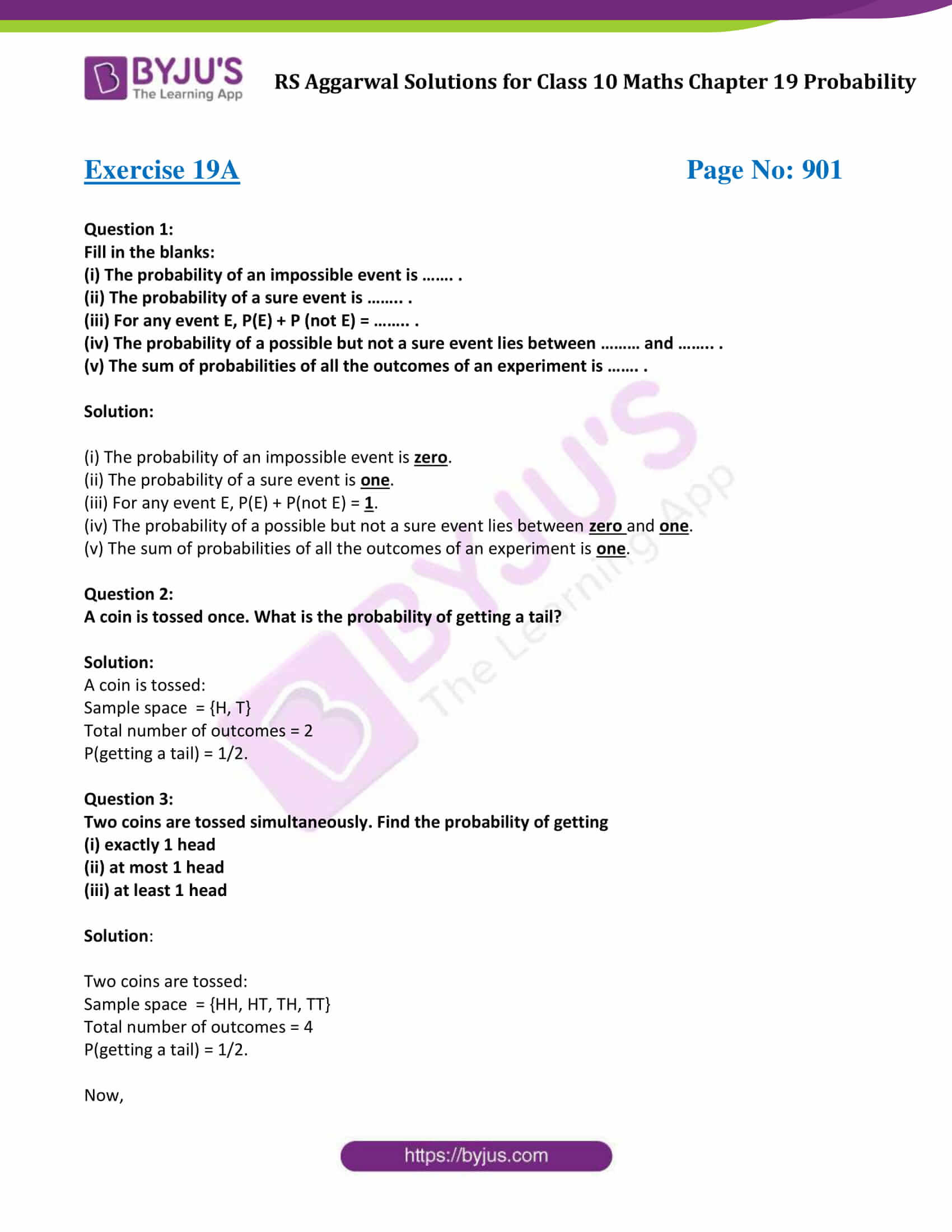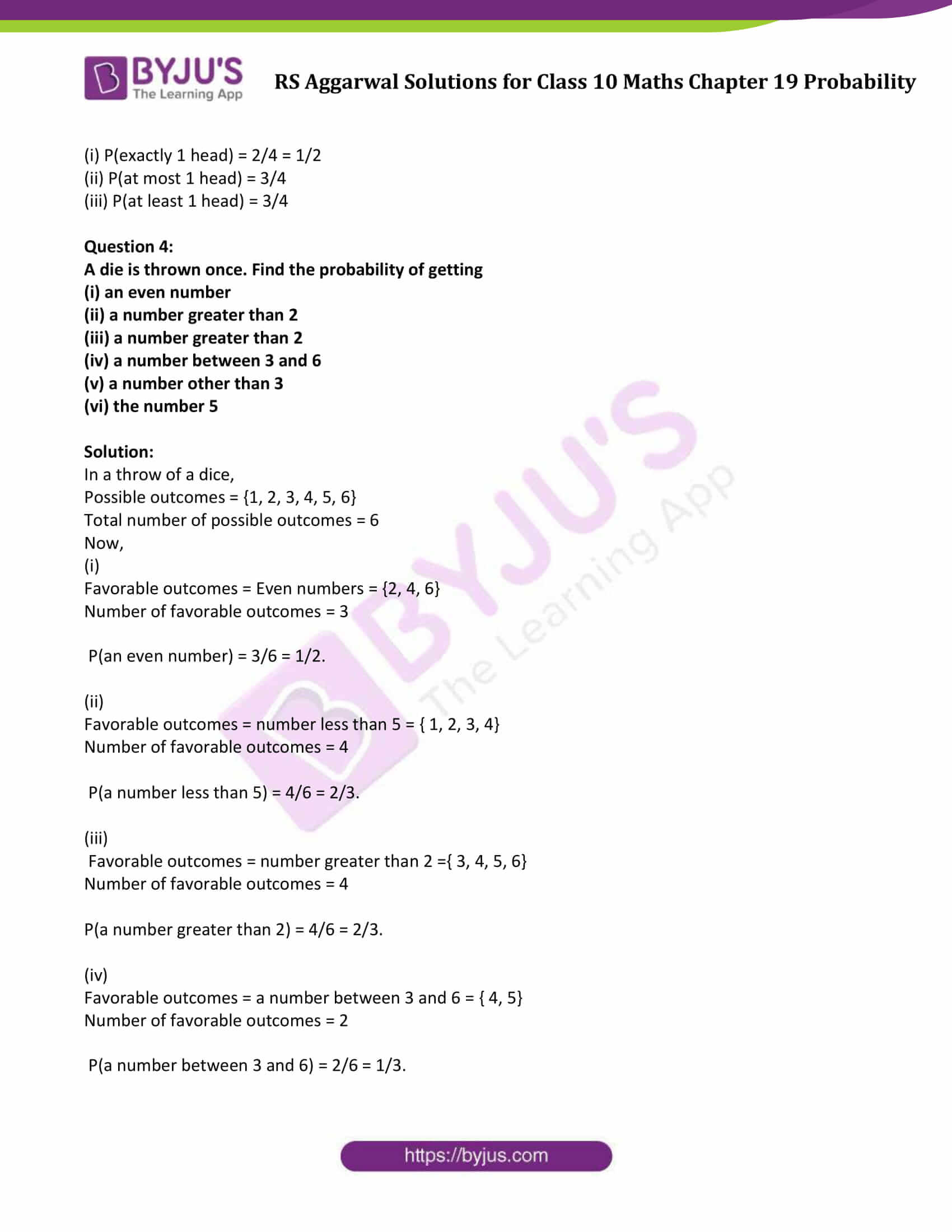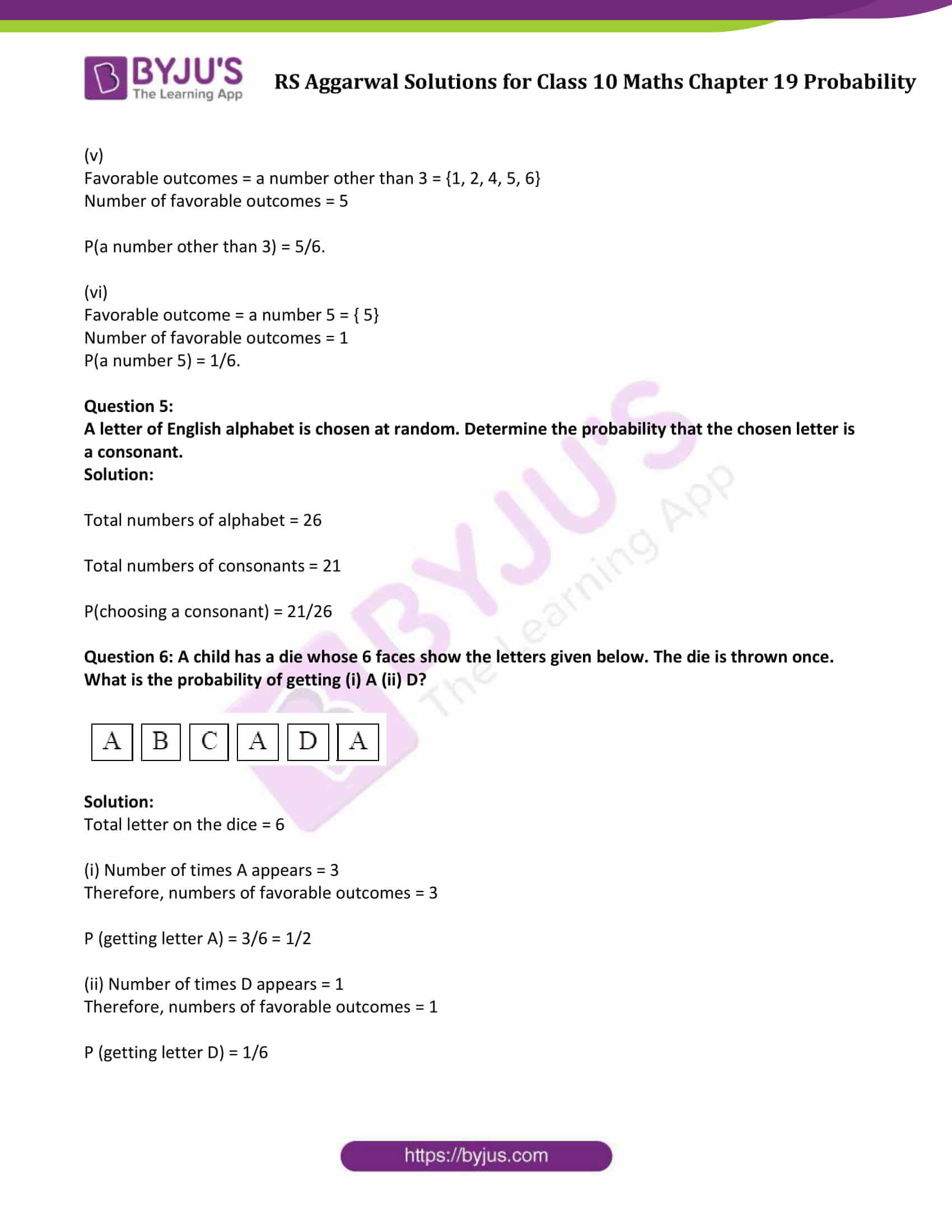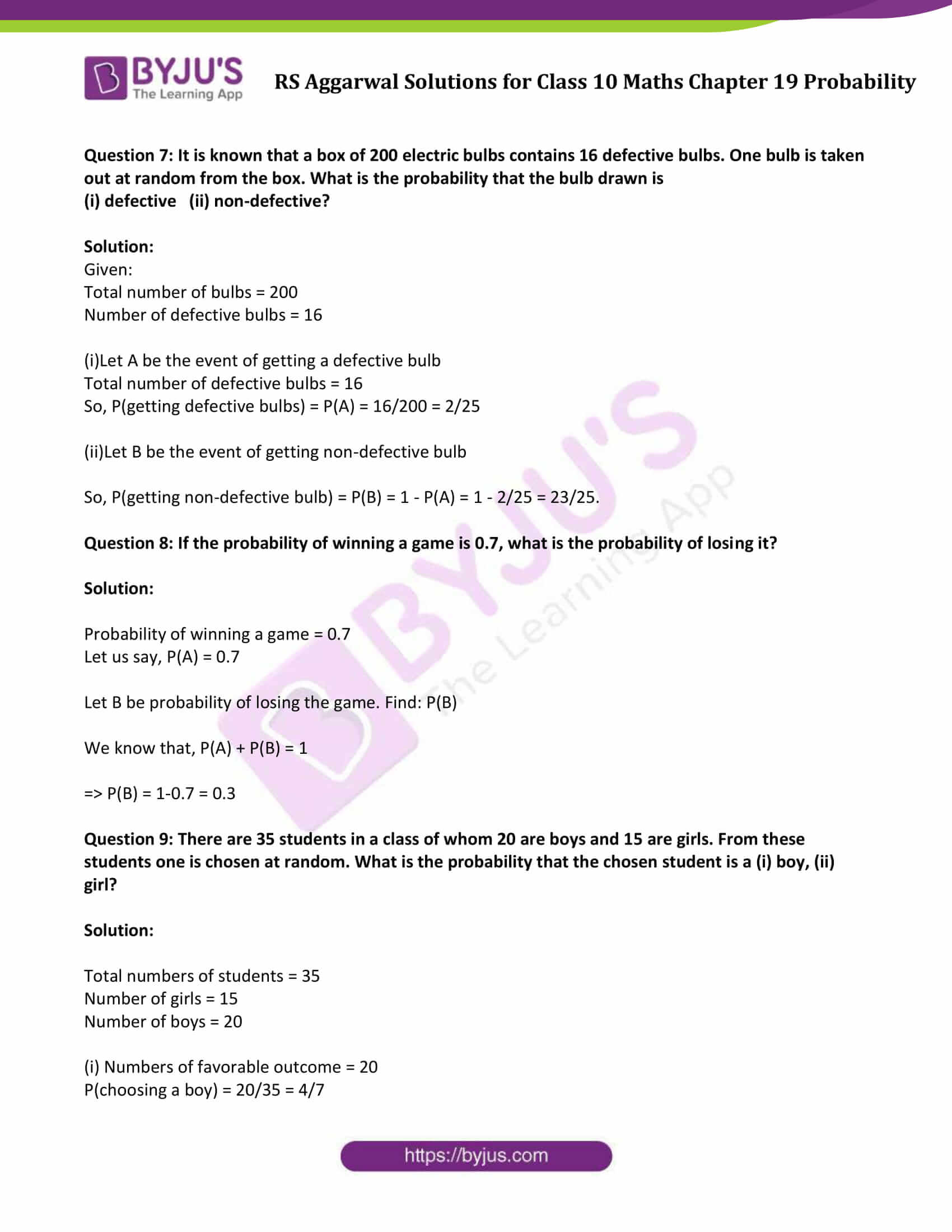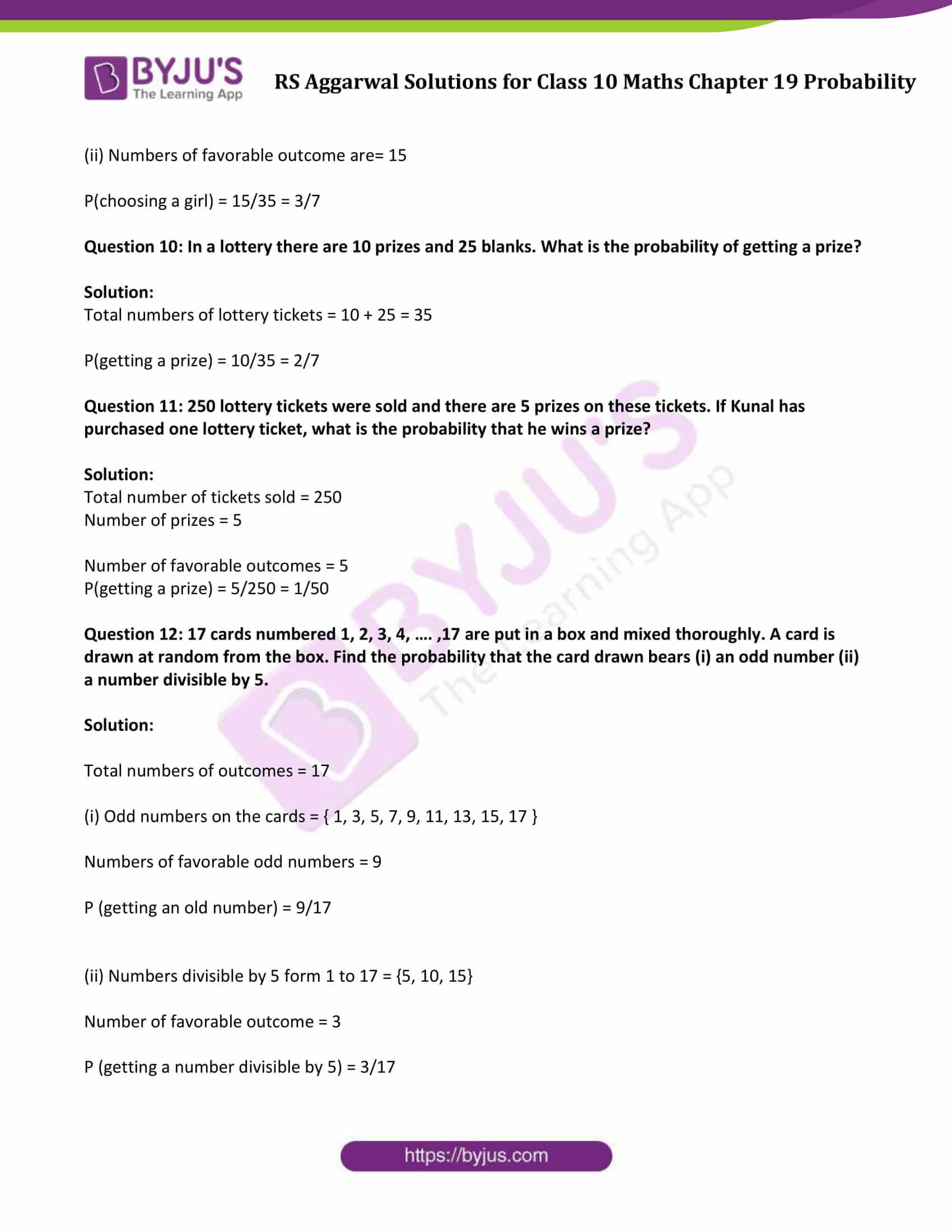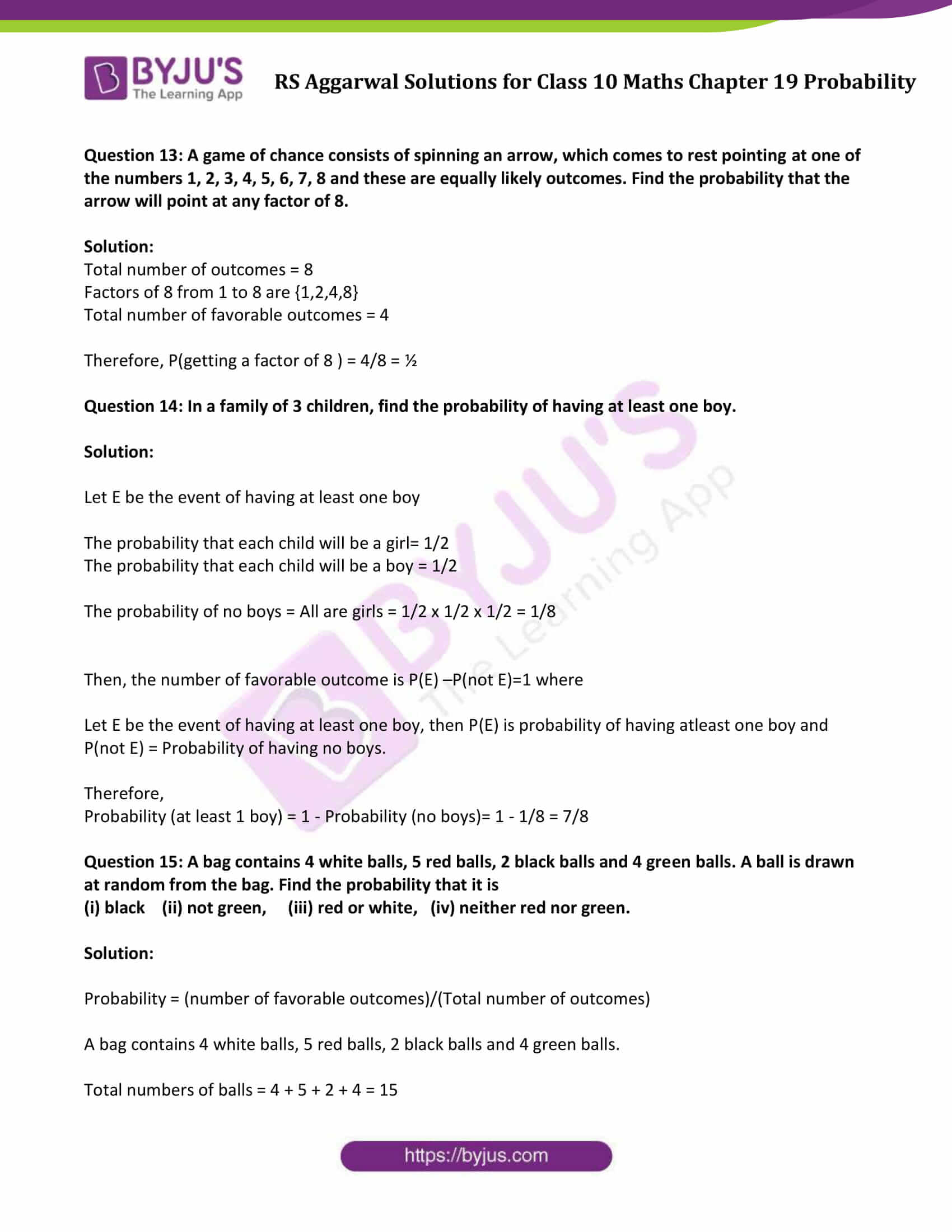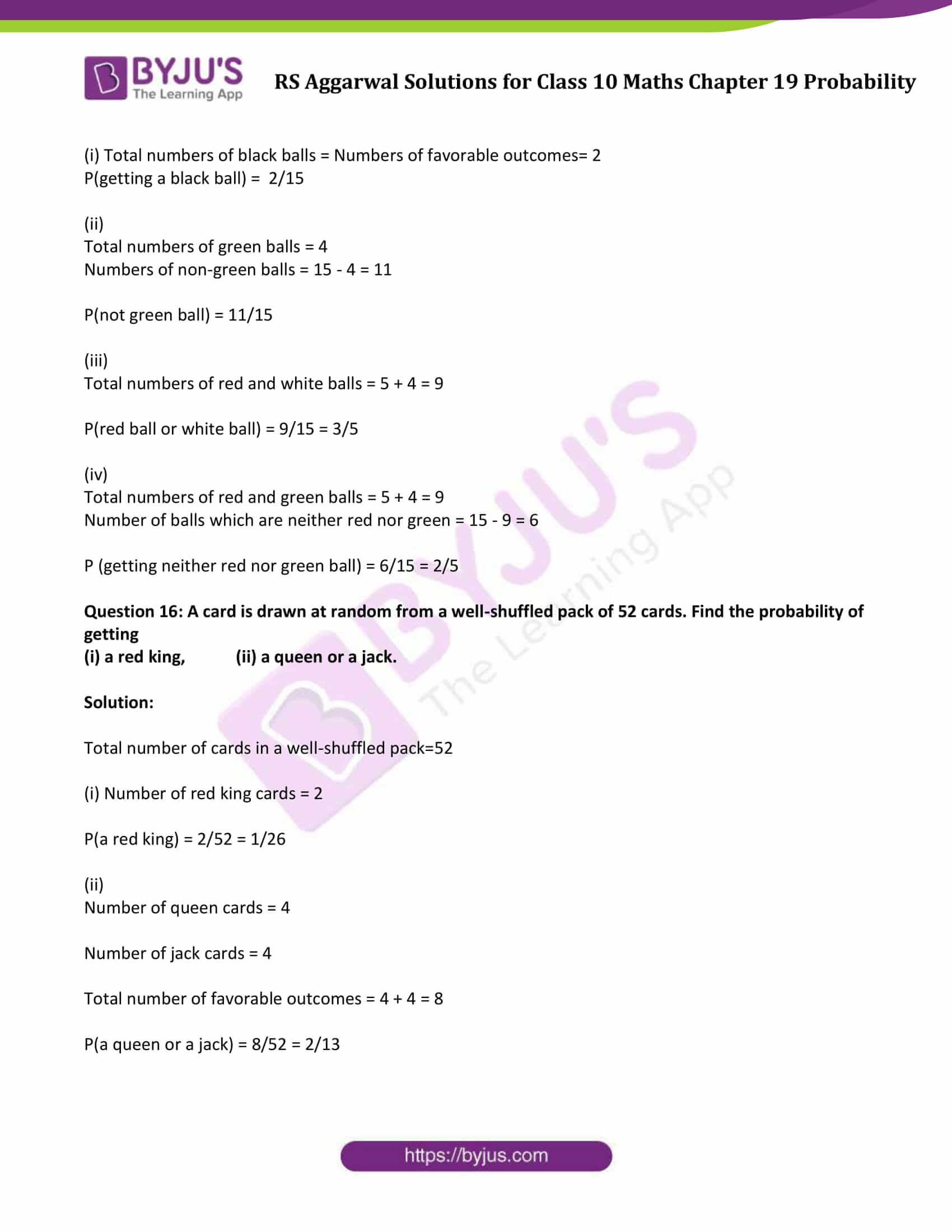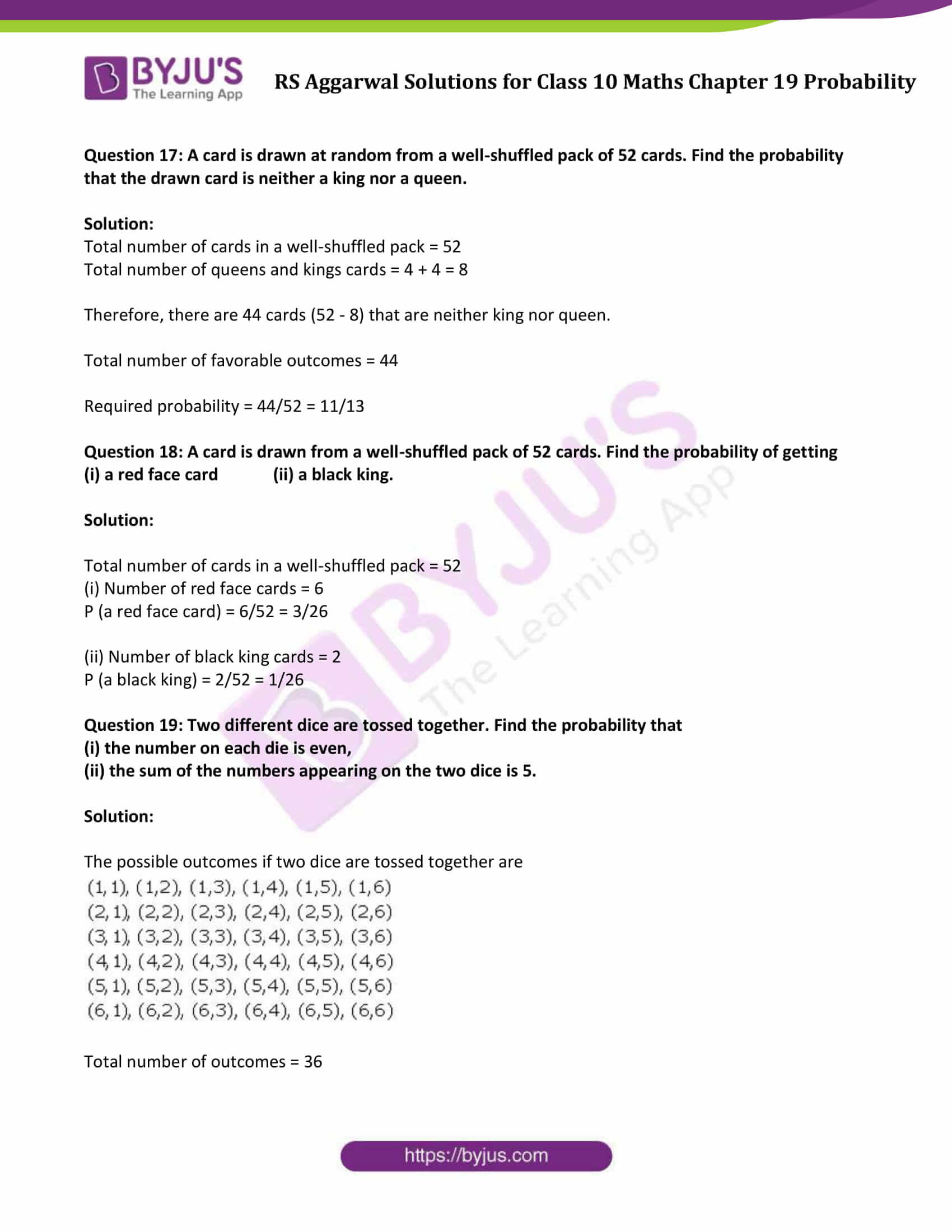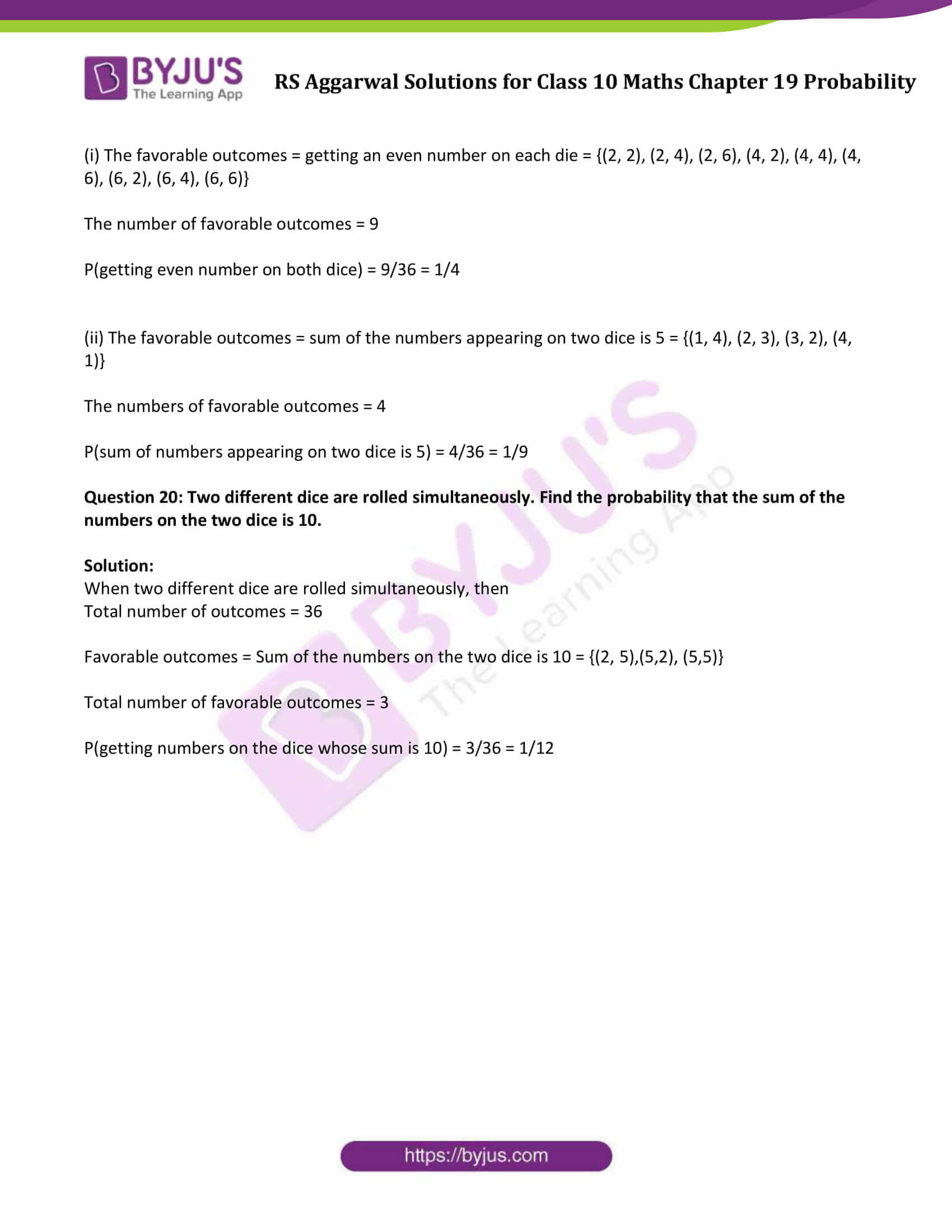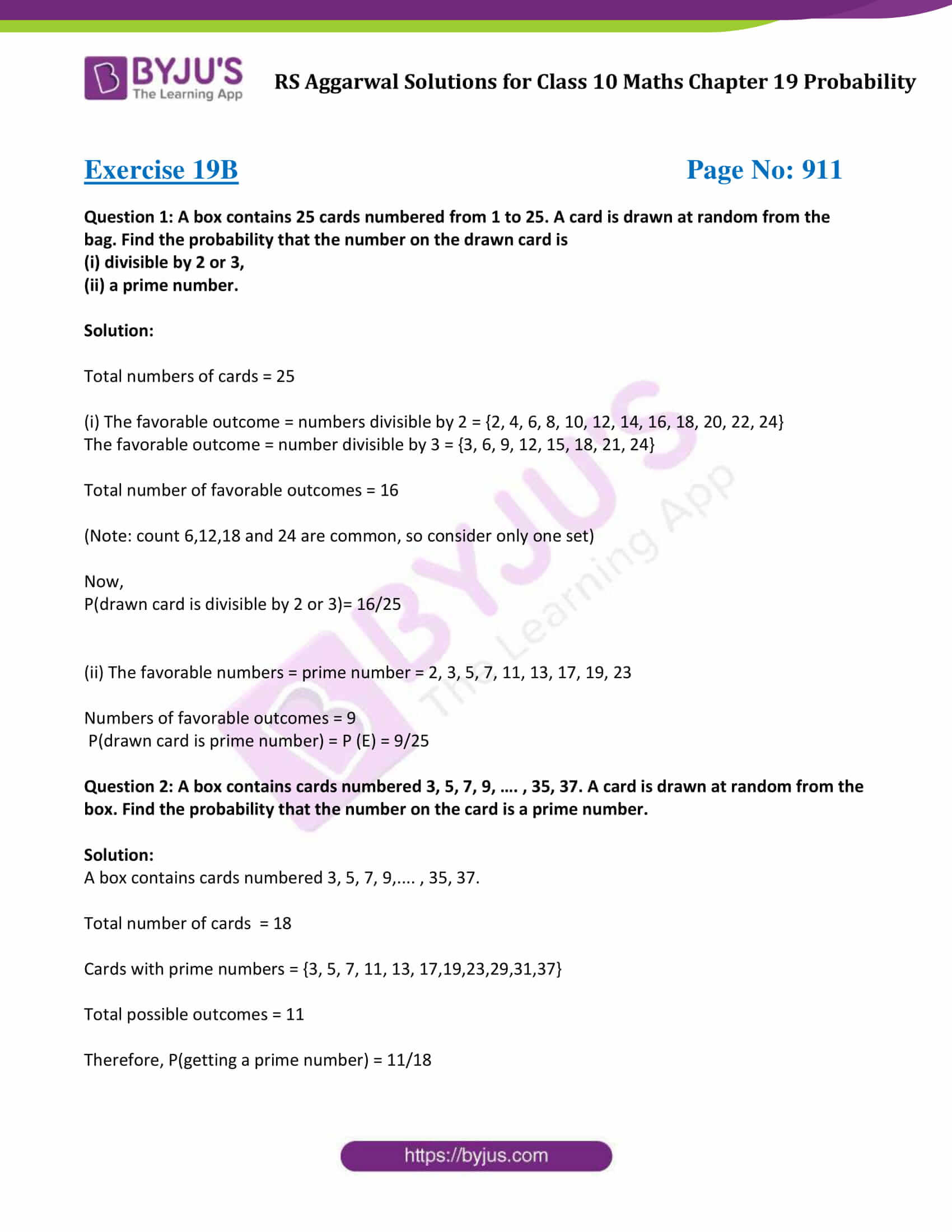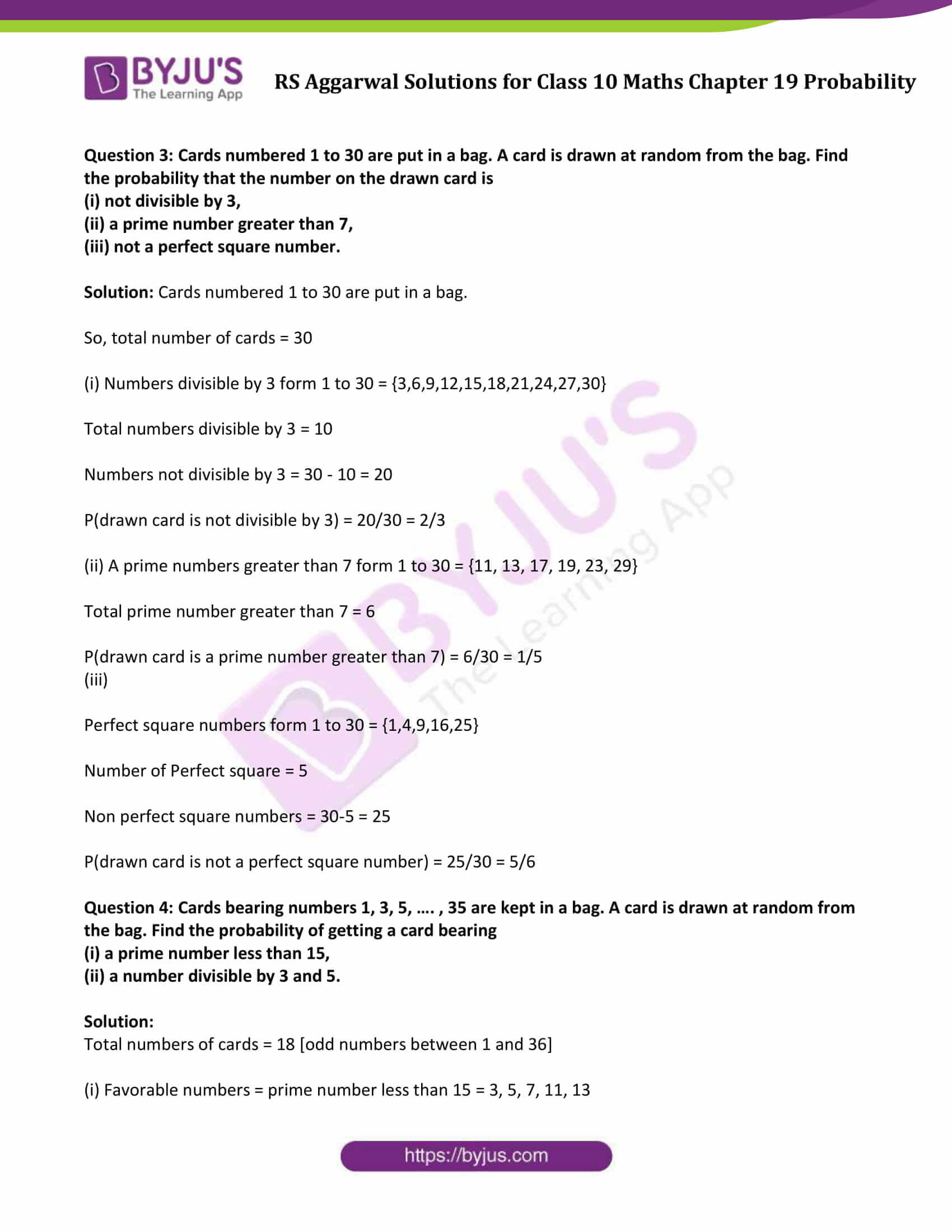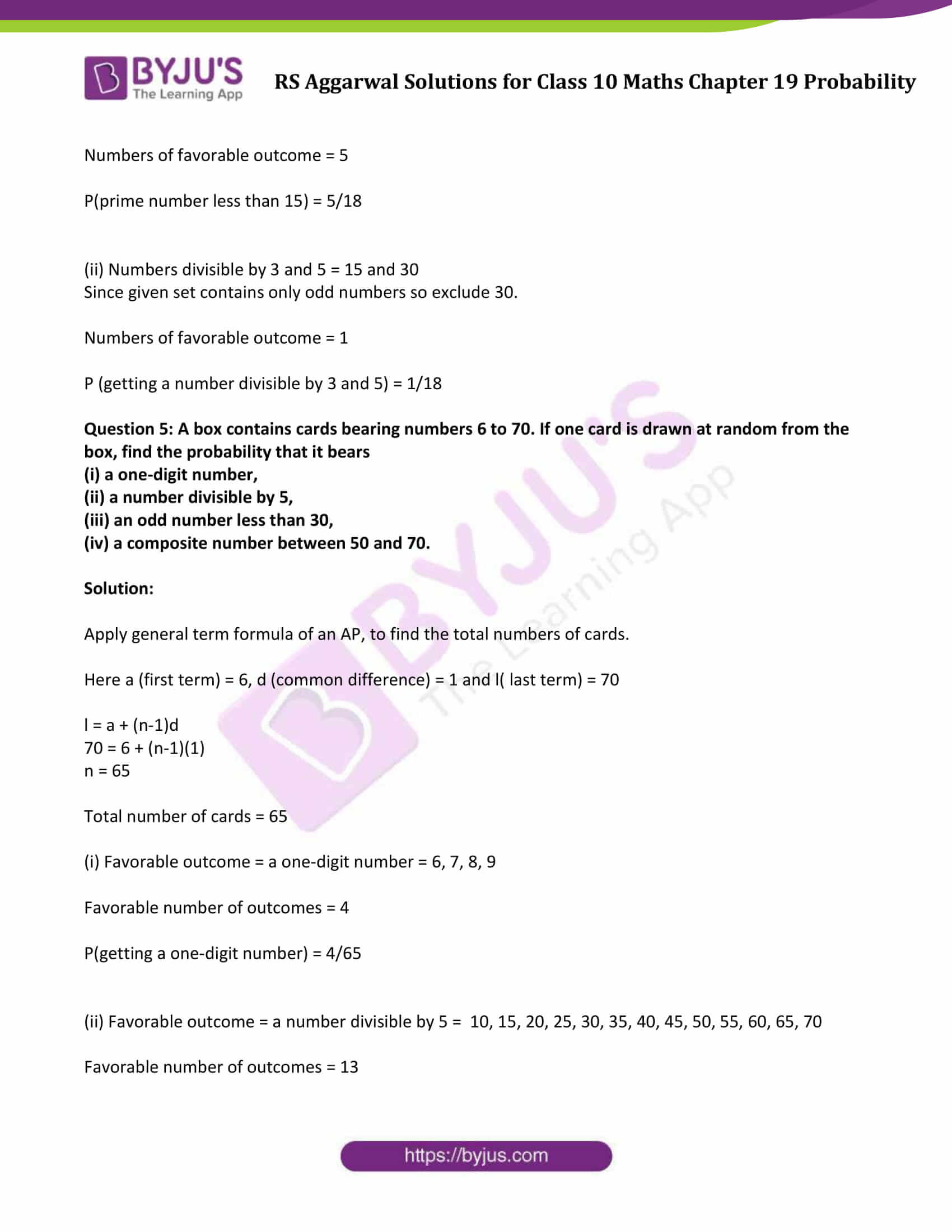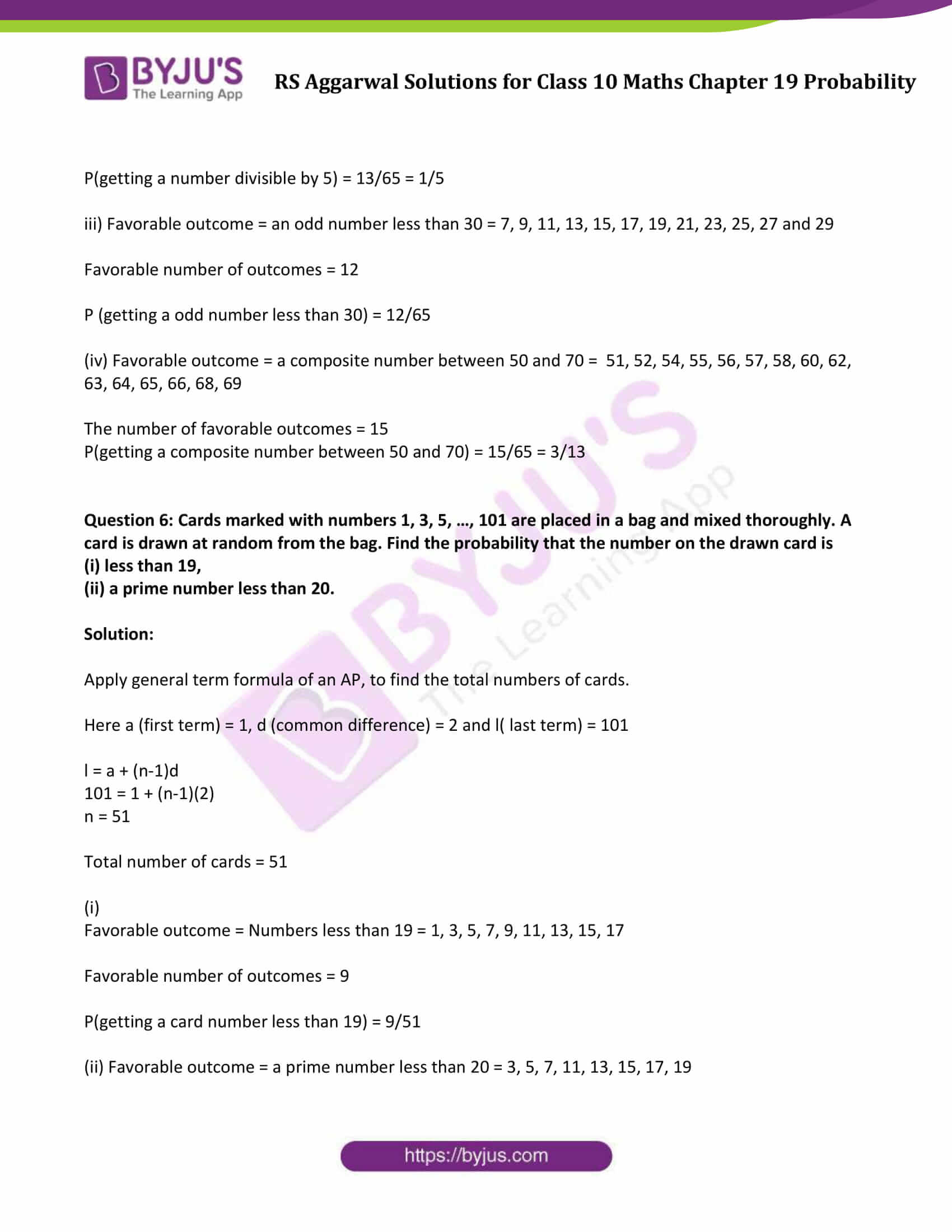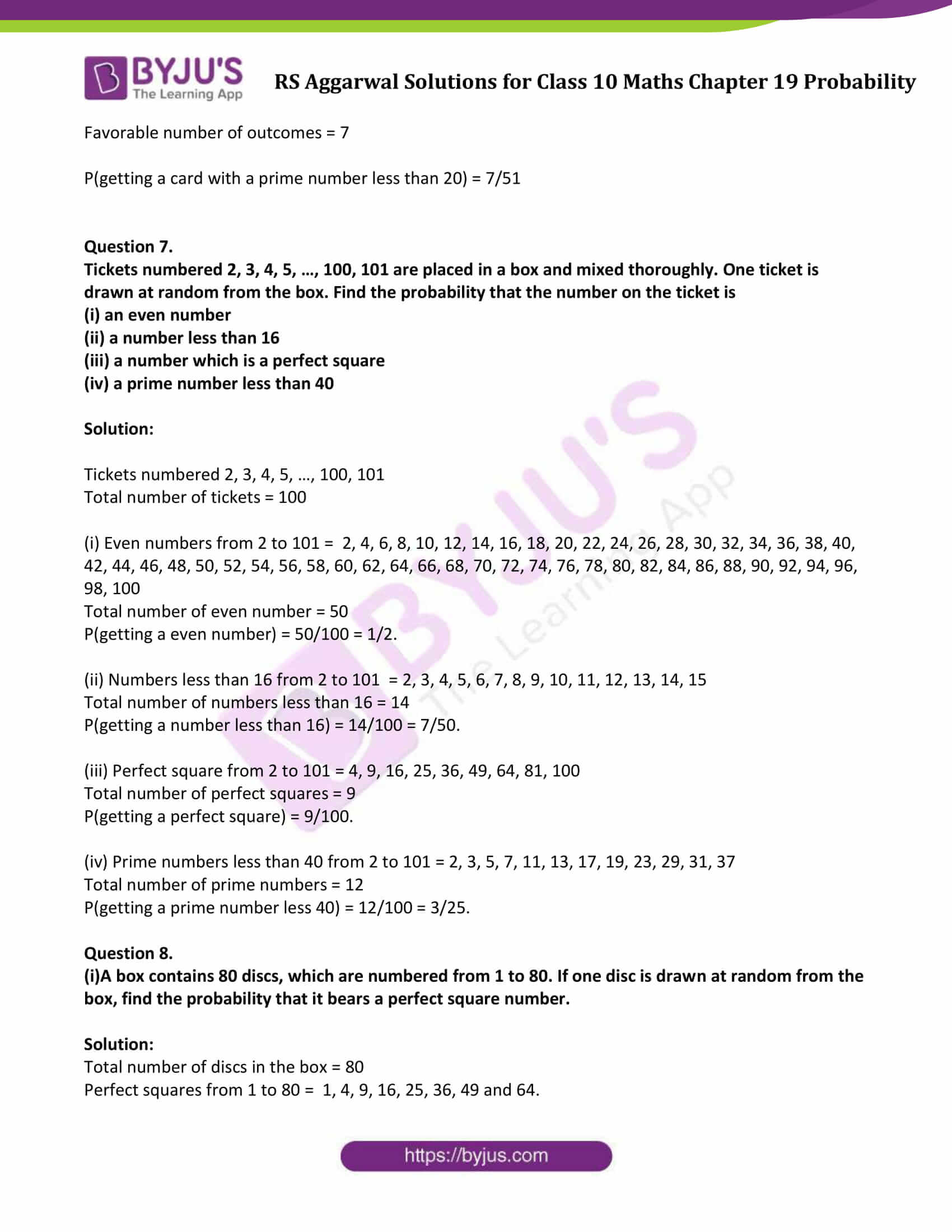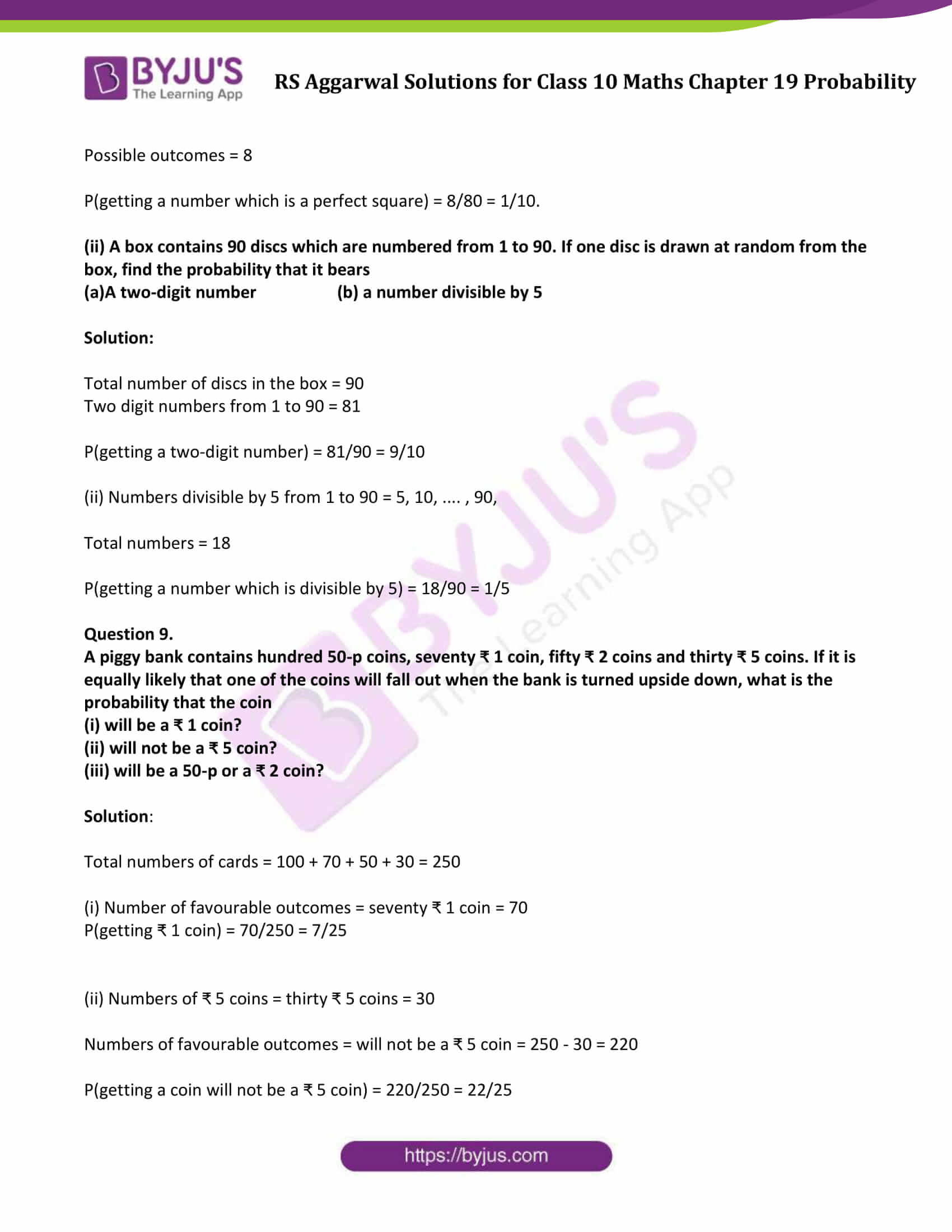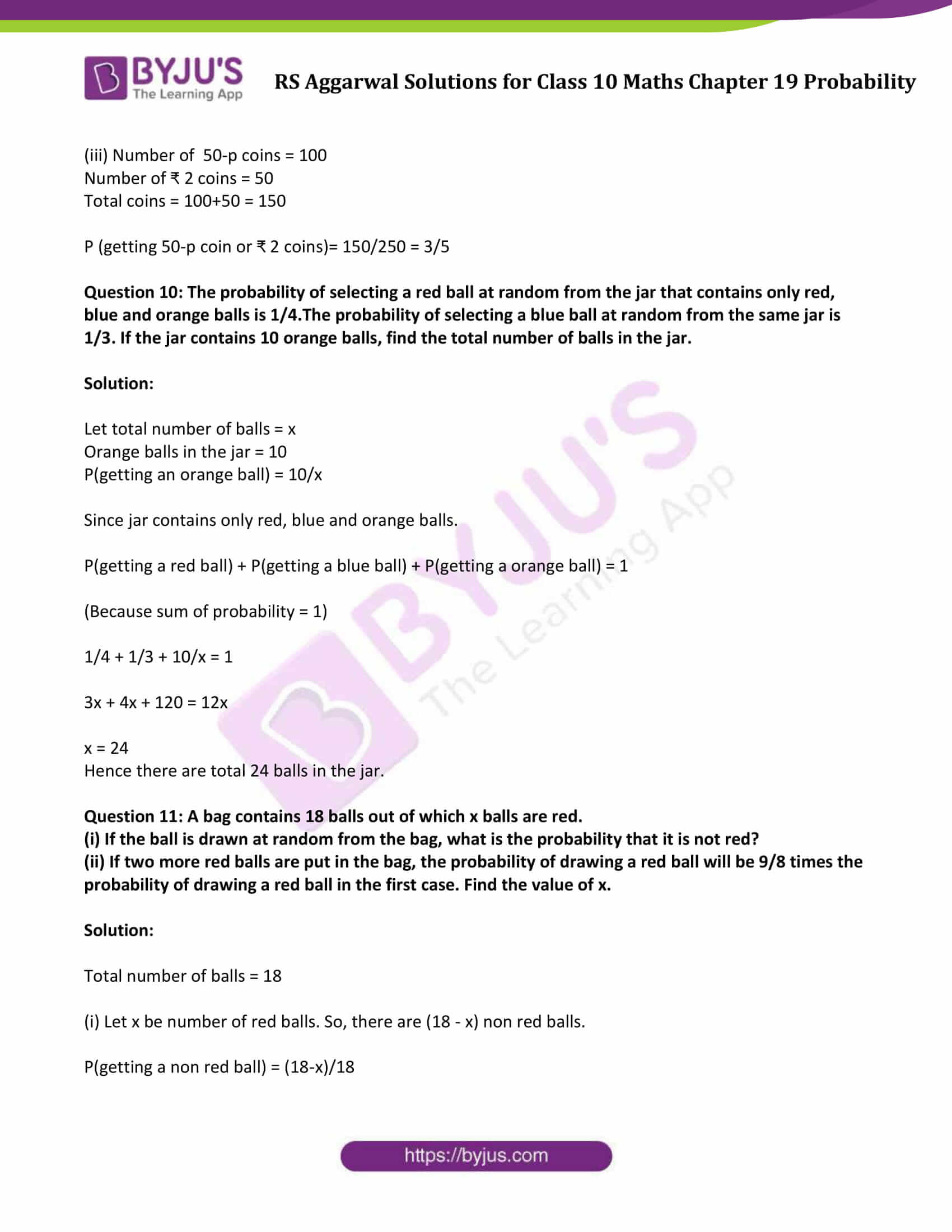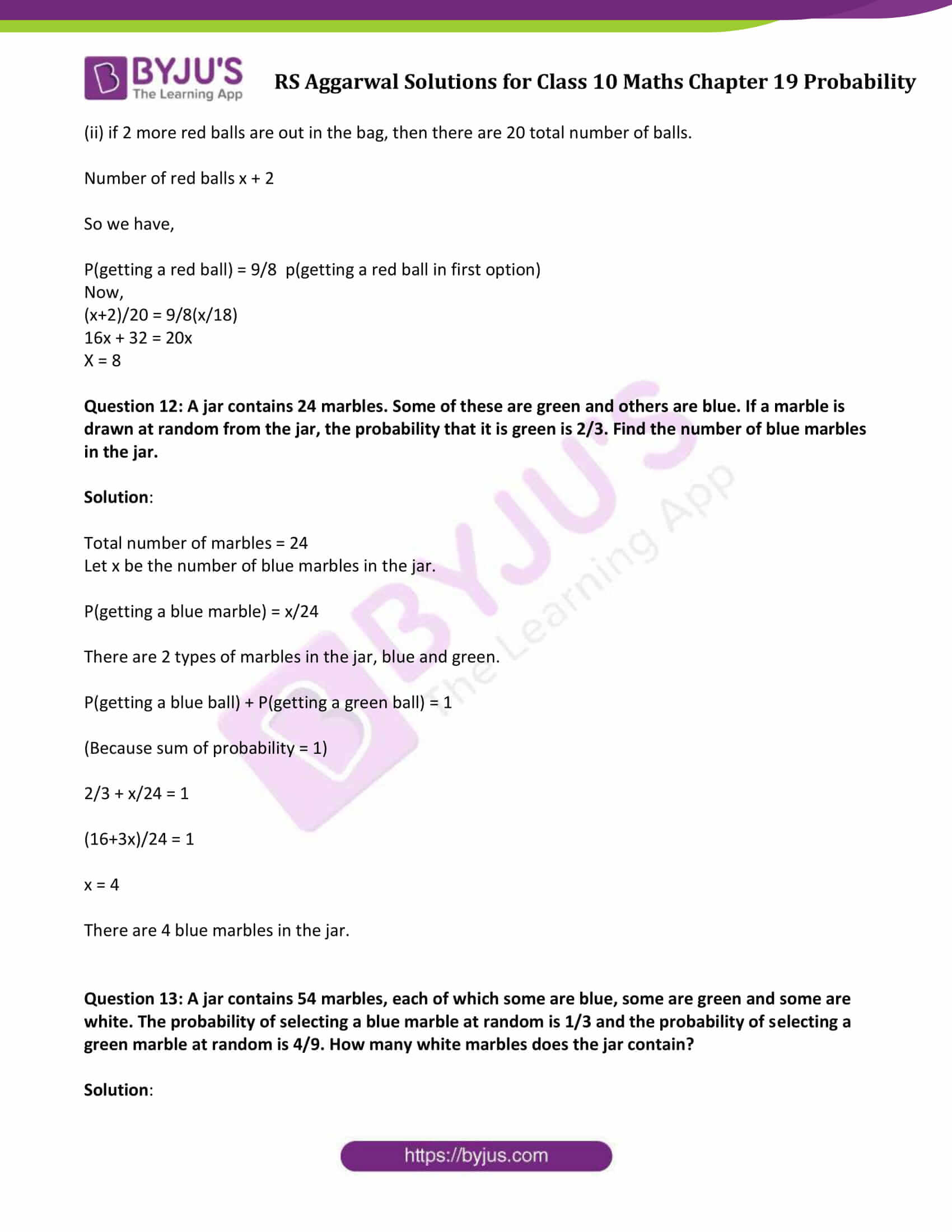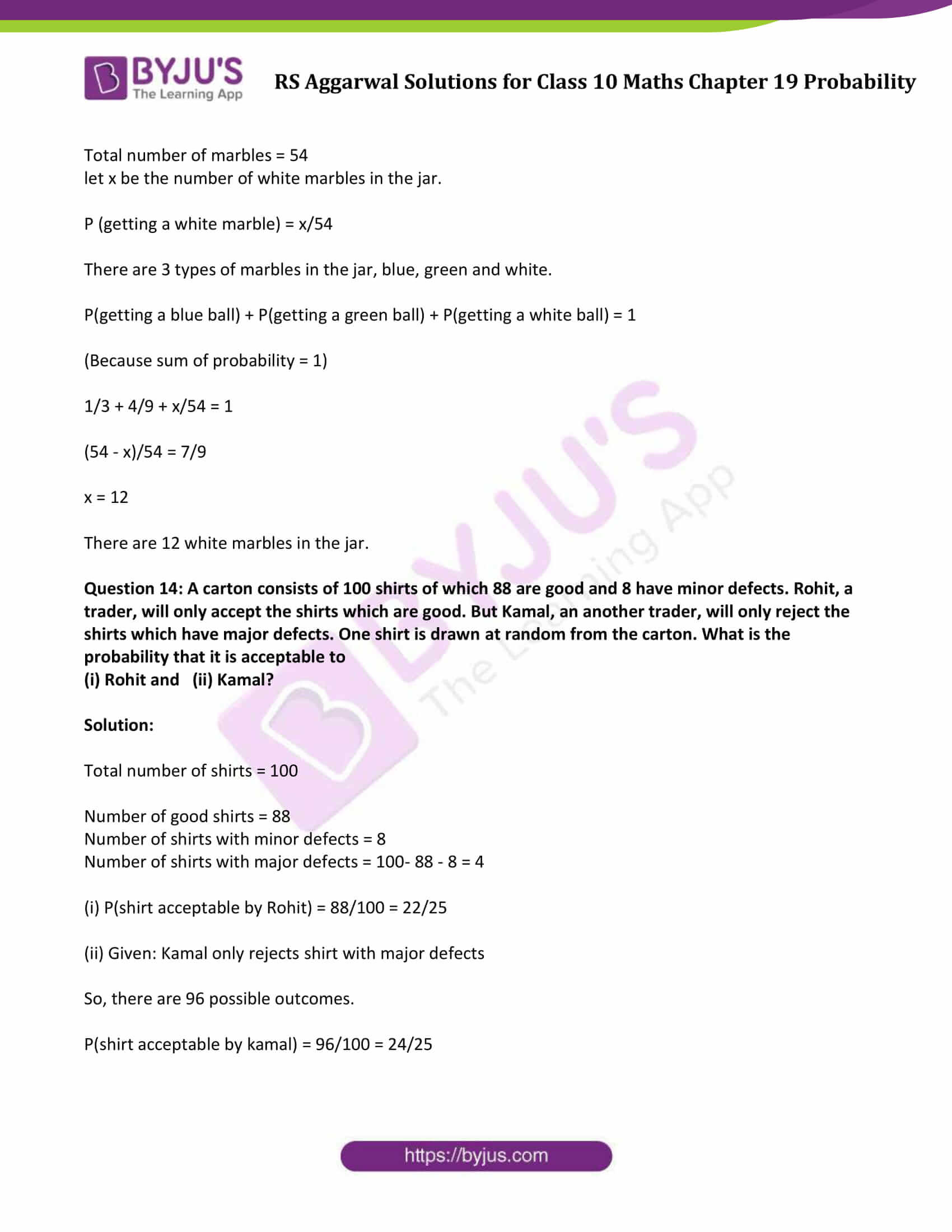### Access Solutions to Maths R S Aggarwal Chapter 19 – Probability

Get detailed solutions for all the questions listed under below exercise:

Exercise 19A Solutions

Exercise 19B Solutions

Exercise 19A

Question 1:

Fill in the blanks:

(i) The probability of an impossible event is ……. .

(ii) The probability of a sure event is …….. .

(iii) For any event E, P(E) + P (not E) = …….. .

(iv) The probability of a possible but not a sure event lies between ……… and …….. .

(v) The sum of probabilities of all the outcomes of an experiment is ……. .

Solution:

(i) The probability of an impossible event is zero.

(ii) The probability of a sure event is one.

(iii) For any event E, P(E) + P(not E) = 1.

(iv) The probability of a possible but not a sure event lies between zero and one.

(v) The sum of probabilities of all the outcomes of an experiment is one.

Question 2:

A coin is tossed once. What is the probability of getting a tail?

Solution:

A coin is tossed:

Sample space = {H, T}

Total number of outcomes = 2

P(getting a tail) = 1/2.

Question 3:

Two coins are tossed simultaneously. Find the probability of getting

Solution:

Two coins are tossed:

Sample space = {HH, HT, TH, TT}

Total number of outcomes = 4

P(getting a tail) = 1/2.

Now,

(i) P(exactly 1 head) = 2/4 = 1/2

(ii) P(at most 1 head) = 3/4

(iii) P(at least 1 head) = 3/4

Question 4:

A die is thrown once. Find the probability of getting

(i) an even number

(ii) a number greater than 2

(iii) a number greater than 2

(iv) a number between 3 and 6

(v) a number other than 3

(vi) the number 5

Solution:

In a throw of a dice,

Possible outcomes = {1, 2, 3, 4, 5, 6}

Total number of possible outcomes = 6

Now,

(i)

Favorable outcomes = Even numbers = {2, 4, 6}

Number of favorable outcomes = 3

P(an even number) = 3/6 = 1/2.

(ii)

Favorable outcomes = number less than 5 = { 1, 2, 3, 4}

Number of favorable outcomes = 4

P(a number less than 5) = 4/6 = 2/3.

(iii)

Favorable outcomes = number greater than 2 ={ 3, 4, 5, 6}

Number of favorable outcomes = 4

P(a number greater than 2) = 4/6 = 2/3.

(iv)

Favorable outcomes = a number between 3 and 6 = { 4, 5}

Number of favorable outcomes = 2

P(a number between 3 and 6) = 2/6 = 1/3.

(v)

Favorable outcomes = a number other than 3 = {1, 2, 4, 5, 6}

Number of favorable outcomes = 5

P(a number other than 3) = 5/6.

(vi)

Favorable outcome = a number 5 = { 5}

Number of favorable outcomes = 1

P(a number 5) = 1/6.

Question 5:

A letter of English alphabet is chosen at random. Determine the probability that the chosen letter is a consonant.

Solution:

Total numbers of alphabet = 26

Total numbers of consonants = 21

P(choosing a consonant) = 21/26

Question 6: A child has a die whose 6 faces show the letters given below. The die is thrown once. What is the probability of getting (i) A (ii) D?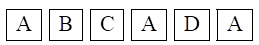Solution:

Total letter on the dice = 6

(i) Number of times A appears = 3

Therefore, numbers of favorable outcomes = 3

P (getting letter A) = 3/6 = 1/2

(ii) Number of times D appears = 1

Therefore, numbers of favorable outcomes = 1

P (getting letter D) = 1/6

Question 7: It is known that a box of 200 electric bulbs contains 16 defective bulbs. One bulb is taken out at random from the box. What is the probability that the bulb drawn is

(i) defective (ii) non-defective?

Solution:

Given:

Total number of bulbs = 200

Number of defective bulbs = 16

(i)Let A be the event of getting a defective bulb

Total number of defective bulbs = 16

So, P(getting defective bulbs) = P(A) = 16/200 = 2/25

(ii)Let B be the event of getting non-defective bulb

So, P(getting non-defective bulb) = P(B) = 1 – P(A) = 1 – 2/25 = 23/25.

Question 8: If the probability of winning a game is 0.7, what is the probability of losing it?

Solution:

Probability of winning a game = 0.7

Let us say, P(A) = 0.7

Let B be probability of losing the game. Find: P(B)

We know that, P(A) + P(B) = 1

=> P(B) = 1-0.7 = 0.3

Question 9: There are 35 students in a class of whom 20 are boys and 15 are girls. From these students one is chosen at random. What is the probability that the chosen student is a (i) boy, (ii) girl?

Solution:

Total numbers of students = 35

Number of girls = 15

Number of boys = 20

(i) Numbers of favorable outcome = 20

P(choosing a boy) = 20/35 = 4/7

(ii) Numbers of favorable outcome are= 15

P(choosing a girl) = 15/35 = 3/7

Question 10: In a lottery there are 10 prizes and 25 blanks. What is the probability of getting a prize?

Solution:

Total numbers of lottery tickets = 10 + 25 = 35

P(getting a prize) = 10/35 = 2/7

Question 11: 250 lottery tickets were sold and there are 5 prizes on these tickets. If Kunal has purchased one lottery ticket, what is the probability that he wins a prize?

Solution:

Total number of tickets sold = 250

Number of prizes = 5

Number of favorable outcomes = 5

P(getting a prize) = 5/250 = 1/50

Question 12: 17 cards numbered 1, 2, 3, 4, …. ,17 are put in a box and mixed thoroughly. A card is drawn at random from the box. Find the probability that the card drawn bears (i) an odd number (ii) a number divisible by 5.

Solution:

Total numbers of outcomes = 17

(i) Odd numbers on the cards = { 1, 3, 5, 7, 9, 11, 13, 15, 17 }

Numbers of favorable odd numbers = 9

P (getting an old number) = 9/17

(ii) Numbers divisible by 5 form 1 to 17 = {5, 10, 15}

Number of favorable outcome = 3

P (getting a number divisible by 5) = 3/17

Question 13: A game of chance consists of spinning an arrow, which comes to rest pointing at one of the numbers 1, 2, 3, 4, 5, 6, 7, 8 and these are equally likely outcomes. Find the probability that the arrow will point at any factor of 8.

Solution:

Total number of outcomes = 8

Factors of 8 from 1 to 8 are {1,2,4,8}

Total number of favorable outcomes = 4

Therefore, P(getting a factor of 8 ) = 4/8 = ½

Question 14: In a family of 3 children, find the probability of having at least one boy.

Solution:

Let E be the event of having at least one boy

The probability that each child will be a girl= 1/2

The probability that each child will be a boy = 1/2

The probability of no boys = All are girls = 1/2 x 1/2 x 1/2 = 1/8

Then, the number of favorable outcome is P(E) –P(not E)=1 where

Let E be the event of having at least one boy, then P(E) is probability of having atleast one boy and

P(not E) = Probability of having no boys.

Therefore,

Probability (at least 1 boy) = 1 – Probability (no boys)= 1 – 1/8 = 7/8

Question 15: A bag contains 4 white balls, 5 red balls, 2 black balls and 4 green balls. A ball is drawn at random from the bag. Find the probability that it is

(i) black (ii) not green, (iii) red or white, (iv) neither red nor green.

Solution:

Probability = (number of favorable outcomes)/(Total number of outcomes)

A bag contains 4 white balls, 5 red balls, 2 black balls and 4 green balls.

Total numbers of balls = 4 + 5 + 2 + 4 = 15

(i) Total numbers of black balls = Numbers of favorable outcomes= 2

P(getting a black ball) = 2/15

(ii)

Total numbers of green balls = 4

Numbers of non-green balls = 15 – 4 = 11

P(not green ball) = 11/15

(iii)

Total numbers of red and white balls = 5 + 4 = 9

P(red ball or white ball) = 9/15 = 3/5

(iv)

Total numbers of red and green balls = 5 + 4 = 9

Number of balls which are neither red nor green = 15 – 9 = 6

P (getting neither red nor green ball) = 6/15 = 2/5

Question 16: A card is drawn at random from a well-shuffled pack of 52 cards. Find the probability of getting

(i) a red king, (ii) a queen or a jack.

Solution:

Total number of cards in a well-shuffled pack=52

(i) Number of red king cards = 2

P(a red king) = 2/52 = 1/26

(ii)

Number of queen cards = 4

Number of jack cards = 4

Total number of favorable outcomes = 4 + 4 = 8

P(a queen or a jack) = 8/52 = 2/13

Question 17: A card is drawn at random from a well-shuffled pack of 52 cards. Find the probability that the drawn card is neither a king nor a queen.

Solution:

Total number of cards in a well-shuffled pack = 52

Total number of queens and kings cards = 4 + 4 = 8

Therefore, there are 44 cards (52 – 8) that are neither king nor queen.

Total number of favorable outcomes = 44

Required probability = 44/52 = 11/13

Question 18: A card is drawn from a well-shuffled pack of 52 cards. Find the probability of getting

(i) a red face card (ii) a black king.

Solution:

Total number of cards in a well-shuffled pack = 52

(i) Number of red face cards = 6

P (a red face card) = 6/52 = 3/26

(ii) Number of black king cards = 2

P (a black king) = 2/52 = 1/26

Question 19: Two different dice are tossed together. Find the probability that

(i) the number on each die is even,

(ii) the sum of the numbers appearing on the two dice is 5.

Solution:

The possible outcomes if two dice are tossed together are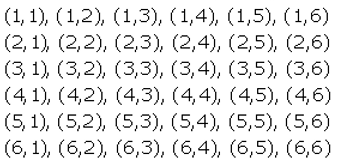Total number of outcomes = 36

(i) The favorable outcomes = getting an even number on each die = {(2, 2), (2, 4), (2, 6), (4, 2), (4, 4), (4, 6), (6, 2), (6, 4), (6, 6)}

The number of favorable outcomes = 9

P(getting even number on both dice) = 9/36 = 1/4

(ii) The favorable outcomes = sum of the numbers appearing on two dice is 5 = {(1, 4), (2, 3), (3, 2), (4, 1)}

The numbers of favorable outcomes = 4

P(sum of numbers appearing on two dice is 5) = 4/36 = 1/9

Question 20: Two different dice are rolled simultaneously. Find the probability that the sum of the numbers on the two dice is 10.

Solution:

When two different dice are rolled simultaneously, then

Total number of outcomes = 36

Favorable outcomes = Sum of the numbers on the two dice is 10 = {(2, 5),(5,2), (5,5)}

Total number of favorable outcomes = 3

P(getting numbers on the dice whose sum is 10) = 3/36 = 1/12

## Exercise 19B

Question 1: A box contains 25 cards numbered from 1 to 25. A card is drawn at random from the bag. Find the probability that the number on the drawn card is

(i) divisible by 2 or 3,

(ii) a prime number.

Solution:

Total numbers of cards = 25

(i) The favorable outcome = numbers divisible by 2 = {2, 4, 6, 8, 10, 12, 14, 16, 18, 20, 22, 24}

The favorable outcome = number divisible by 3 = {3, 6, 9, 12, 15, 18, 21, 24}

Total number of favorable outcomes = 16

(Note: count 6,12,18 and 24 are common, so consider only one set)

Now,

P(drawn card is divisible by 2 or 3)= 16/25

(ii) The favorable numbers = prime number = 2, 3, 5, 7, 11, 13, 17, 19, 23

Numbers of favorable outcomes = 9

P(drawn card is prime number) = P (E) = 9/25

Question 2: A box contains cards numbered 3, 5, 7, 9, …. , 35, 37. A card is drawn at random from the box. Find the probability that the number on the card is a prime number.

Solution:

A box contains cards numbered 3, 5, 7, 9,…. , 35, 37.

Total number of cards = 18

Cards with prime numbers = {3, 5, 7, 11, 13, 17,19,23,29,31,37}

Total possible outcomes = 11

Therefore, P(getting a prime number) = 11/18

Question 3: Cards numbered 1 to 30 are put in a bag. A card is drawn at random from the bag. Find the probability that the number on the drawn card is

(i) not divisible by 3,

(ii) a prime number greater than 7,

(iii) not a perfect square number.

Solution: Cards numbered 1 to 30 are put in a bag.

So, total number of cards = 30

(i) Numbers divisible by 3 form 1 to 30 = {3,6,9,12,15,18,21,24,27,30}

Total numbers divisible by 3 = 10

Numbers not divisible by 3 = 30 – 10 = 20

P(drawn card is not divisible by 3) = 20/30 = 2/3

(ii) A prime numbers greater than 7 form 1 to 30 = {11, 13, 17, 19, 23, 29}

Total prime number greater than 7 = 6

P(drawn card is a prime number greater than 7) = 6/30 = 1/5

(iii)

Perfect square numbers form 1 to 30 = {1,4,9,16,25}

Number of Perfect square = 5

Non perfect square numbers = 30-5 = 25

P(drawn card is not a perfect square number) = 25/30 = 5/6

Question 4: Cards bearing numbers 1, 3, 5, …. , 35 are kept in a bag. A card is drawn at random from the bag. Find the probability of getting a card bearing

(i) a prime number less than 15,

(ii) a number divisible by 3 and 5.

Solution:

Total numbers of cards = 18 [odd numbers between 1 and 36]

(i) Favorable numbers = prime number less than 15 = 3, 5, 7, 11, 13

Numbers of favorable outcome = 5

P(prime number less than 15) = 5/18

(ii) Numbers divisible by 3 and 5 = 15 and 30

Since given set contains only odd numbers so exclude 30.

Numbers of favorable outcome = 1

P (getting a number divisible by 3 and 5) = 1/18

Question 5: A box contains cards bearing numbers 6 to 70. If one card is drawn at random from the box, find the probability that it bears

(i) a one-digit number,

(ii) a number divisible by 5,

(iii) an odd number less than 30,

(iv) a composite number between 50 and 70.

Solution:

Apply general term formula of an AP, to find the total numbers of cards.

Here a (first term) = 6, d (common difference) = 1 and l( last term) = 70

l = a + (n-1)d

70 = 6 + (n-1)(1)

n = 65

Total number of cards = 65

(i) Favorable outcome = a one-digit number = 6, 7, 8, 9

Favorable number of outcomes = 4

P(getting a one-digit number) = 4/65

(ii) Favorable outcome = a number divisible by 5 = 10, 15, 20, 25, 30, 35, 40, 45, 50, 55, 60, 65, 70

Favorable number of outcomes = 13

P(getting a number divisible by 5) = 13/65 = 1/5

iii) Favorable outcome = an odd number less than 30 = 7, 9, 11, 13, 15, 17, 19, 21, 23, 25, 27 and 29

Favorable number of outcomes = 12

P (getting a odd number less than 30) = 12/65

(iv) Favorable outcome = a composite number between 50 and 70 = 51, 52, 54, 55, 56, 57, 58, 60, 62, 63, 64, 65, 66, 68, 69

The number of favorable outcomes = 15

P(getting a composite number between 50 and 70) = 15/65 = 3/13

Question 6: Cards marked with numbers 1, 3, 5, …, 101 are placed in a bag and mixed thoroughly. A card is drawn at random from the bag. Find the probability that the number on the drawn card is

(i) less than 19,

(ii) a prime number less than 20.

Solution:

Apply general term formula of an AP, to find the total numbers of cards.

Here a (first term) = 1, d (common difference) = 2 and l( last term) = 101

l = a + (n-1)d

101 = 1 + (n-1)(2)

n = 51

Total number of cards = 51

(i)

Favorable outcome = Numbers less than 19 = 1, 3, 5, 7, 9, 11, 13, 15, 17

Favorable number of outcomes = 9

P(getting a card number less than 19) = 9/51

(ii) Favorable outcome = a prime number less than 20 = 3, 5, 7, 11, 13, 15, 17, 19

Favorable number of outcomes = 7

P(getting a card with a prime number less than 20) = 7/51

Question 7.

Tickets numbered 2, 3, 4, 5, …, 100, 101 are placed in a box and mixed thoroughly. One ticket is drawn at random from the box. Find the probability that the number on the ticket is

(i) an even number

(ii) a number less than 16

(iii) a number which is a perfect square

(iv) a prime number less than 40

Solution:

Tickets numbered 2, 3, 4, 5, …, 100, 101

Total number of tickets = 100

(i) Even numbers from 2 to 101 = 2, 4, 6, 8, 10, 12, 14, 16, 18, 20, 22, 24, 26, 28, 30, 32, 34, 36, 38, 40, 42, 44, 46, 48, 50, 52, 54, 56, 58, 60, 62, 64, 66, 68, 70, 72, 74, 76, 78, 80, 82, 84, 86, 88, 90, 92, 94, 96, 98, 100

Total number of even number = 50

P(getting a even number) = 50/100 = 1/2.

(ii) Numbers less than 16 from 2 to 101 = 2, 3, 4, 5, 6, 7, 8, 9, 10, 11, 12, 13, 14, 15

Total number of numbers less than 16 = 14

P(getting a number less than 16) = 14/100 = 7/50.

(iii) Perfect square from 2 to 101 = 4, 9, 16, 25, 36, 49, 64, 81, 100

Total number of perfect squares = 9

P(getting a perfect square) = 9/100.

(iv) Prime numbers less than 40 from 2 to 101 = 2, 3, 5, 7, 11, 13, 17, 19, 23, 29, 31, 37

Total number of prime numbers = 12

P(getting a prime number less 40) = 12/100 = 3/25.

Question 8.

(i)A box contains 80 discs, which are numbered from 1 to 80. If one disc is drawn at random from the box, find the probability that it bears a perfect square number.

Solution:

Total number of discs in the box = 80

Perfect squares from 1 to 80 = 1, 4, 9, 16, 25, 36, 49 and 64.

Possible outcomes = 8

P(getting a number which is a perfect square) = 8/80 = 1/10.

(ii) A box contains 90 discs which are numbered from 1 to 90. If one disc is drawn at random from the box, find the probability that it bears

(a)A two-digit number (b) a number divisible by 5

Solution:

Total number of discs in the box = 90

Two digit numbers from 1 to 90 = 81

P(getting a two-digit number) = 81/90 = 9/10

(ii) Numbers divisible by 5 from 1 to 90 = 5, 10, …. , 90,

Total numbers = 18

P(getting a number which is divisible by 5) = 18/90 = 1/5

Question 9.

A piggy bank contains hundred 50-p coins, seventy ₹ 1 coin, fifty ₹ 2 coins and thirty ₹ 5 coins. If it is equally likely that one of the coins will fall out when the bank is turned upside down, what is the probability that the coin

(i) will be a ₹ 1 coin?

(ii) will not be a ₹ 5 coin?

(iii) will be a 50-p or a ₹ 2 coin?

Solution:

Total numbers of cards = 100 + 70 + 50 + 30 = 250

(i) Number of favourable outcomes = seventy ₹ 1 coin = 70

P(getting ₹ 1 coin) = 70/250 = 7/25

(ii) Numbers of ₹ 5 coins = thirty ₹ 5 coins = 30

Numbers of favourable outcomes = will not be a ₹ 5 coin = 250 – 30 = 220

P(getting a coin will not be a ₹ 5 coin) = 220/250 = 22/25

(iii) Number of 50-p coins = 100

Number of ₹ 2 coins = 50

Total coins = 100+50 = 150

P (getting 50-p coin or ₹ 2 coins)= 150/250 = 3/5

Question 10: The probability of selecting a red ball at random from the jar that contains only red, blue and orange balls is 1/4.The probability of selecting a blue ball at random from the same jar is 1/3. If the jar contains 10 orange balls, find the total number of balls in the jar.

Solution:

Let total number of balls = x

Orange balls in the jar = 10

P(getting an orange ball) = 10/x

Since jar contains only red, blue and orange balls.

P(getting a red ball) + P(getting a blue ball) + P(getting a orange ball) = 1

(Because sum of probability = 1)

1/4 + 1/3 + 10/x = 1

3x + 4x + 120 = 12x

x = 24

Hence there are total 24 balls in the jar.

Question 11: A bag contains 18 balls out of which x balls are red.

(i) If the ball is drawn at random from the bag, what is the probability that it is not red?

(ii) If two more red balls are put in the bag, the probability of drawing a red ball will be 9/8 times the probability of drawing a red ball in the first case. Find the value of x.

Solution:

Total number of balls = 18

(i) Let x be number of red balls. So, there are (18 – x) non red balls.

P(getting a non red ball) = (18-x)/18

(ii) if 2 more red balls are out in the bag, then there are 20 total number of balls.

Number of red balls x + 2

So we have,

P(getting a red ball) = 9/8 p(getting a red ball in first option)

Now,

(x+2)/20 = 9/8(x/18)

16x + 32 = 20x

X = 8

Question 12: A jar contains 24 marbles. Some of these are green and others are blue. If a marble is drawn at random from the jar, the probability that it is green is 2/3. Find the number of blue marbles in the jar.

Solution:

Total number of marbles = 24

Let x be the number of blue marbles in the jar.

P(getting a blue marble) = x/24

There are 2 types of marbles in the jar, blue and green.

P(getting a blue ball) + P(getting a green ball) = 1

(Because sum of probability = 1)

2/3 + x/24 = 1

(16+3x)/24 = 1

x = 4

There are 4 blue marbles in the jar.

Question 13: A jar contains 54 marbles, each of which some are blue, some are green and some are white. The probability of selecting a blue marble at random is 1/3 and the probability of selecting a green marble at random is 4/9. How many white marbles does the jar contain?

Solution:

Total number of marbles = 54

let x be the number of white marbles in the jar.

P (getting a white marble) = x/54

There are 3 types of marbles in the jar, blue, green and white.

P(getting a blue ball) + P(getting a green ball) + P(getting a white ball) = 1

(Because sum of probability = 1)

1/3 + 4/9 + x/54 = 1

(54 – x)/54 = 7/9

x = 12

There are 12 white marbles in the jar.

Question 14: A carton consists of 100 shirts of which 88 are good and 8 have minor defects. Rohit, a trader, will only accept the shirts which are good. But Kamal, an another trader, will only reject the shirts which have major defects. One shirt is drawn at random from the carton. What is the probability that it is acceptable to

(i) Rohit and (ii) Kamal?

Solution:

Total number of shirts = 100

Number of good shirts = 88

Number of shirts with minor defects = 8

Number of shirts with major defects = 100- 88 – 8 = 4

(i) P(shirt acceptable by Rohit) = 88/100 = 22/25

(ii) Given: Kamal only rejects shirt with major defects

So, there are 96 possible outcomes.

P(shirt acceptable by kamal) = 96/100 = 24/25

## R S Aggarwal Solutions for Chapter 19 Probability

In this chapter students will study important concepts on Probability as listed below:

• Probability Introduction

Probability is a concept which numerically measures the degree of certainty of the occurrence of events.

Experiment: An operation which can produce some well-defined outcomes

Random Experiment: An experiment in which all possible outcomes are known, and the exact outcome cannot be predicted in advance, is called a random experiment

• Possible outcomes in various experiments
• Equally likely terms
• Probability of occurrence of an event.
• Word Problems in real-life situations

### Key Features of R S Aggarwal Solutions for Class 10 Maths Chapter 19 Probability

1. R S Aggarwal Solutions will be useful for Olympiads, board exams, and other competitive exams.

2. Easy for quick revision.

3. Prepared based on the latest CBSE syllabus by subject experts.

4. Provides comprehensive answers to all the questions.